• 2019-04-07 16:50:43

## 线性规划-pulp-复杂矩阵

### 1. 简介-线性规划

在数学中，线性规划（Linear Programming，简称LP）特指目标函数和约束条件皆为线性的最优化问题。线性规划是最优化问题中的一个重要领域。在作业研究中所面临的许多实际问题都可以用线性规划来处理，特别是某些特殊情况。最简单情况就，解线性方程组。

举个最简单的例子:

工厂生产A和B两种物品，需要原料配比分别是1：2：1和2：1：3.

现有原料是100，40，8。A和B的价格分别是5和10。

请问如何分配原材料才能获益最大？

求解一个线性方程组即可

### 2. pulp 包

• Python中有很多的工具可以解决线性规划问题。由于pulp因其常用和方便性，故这里介绍pulp包的使用。pulp能够解决大部分的线性规划问题，如""整数规划（Integer Program）、01规划（Binary Program）还是混合整数线性规划（MILP），都能很好的解决。

• pulp的github网址和官方文档，说实话官方文档做的并不好，但是里面介绍的例子还是很好的，如果只做最简单的线性规划可以看看学习一下。

• 安装：按照一般套路pip install pulp 即可。

### 3. pulp使用流程

• 获得问题的描述：求解最大值还是最小值，这个要明确了。输入是的数据是什么。
• 确定需要的决策变量：需要求解的变量，定义好，大小，数据类型。
• 制定约束条件：决策变量和输入数据之间存在什么样的约束条件。
• 输入数据，求解得到结果。

那个实际例子来说明：

1. 获得问题的描述：我们有矩阵X和矩阵Y，大小是1008*6。如图最上面的例子：是1008个产品，在6+6个指标上的需求。拆分成6+6两部分可以说明更加复杂的关系，以便更具有扩展性。

目标，我们要求最大值： max ⁡ ∑ j = 1 n φ j = ∑ j = 1 n ∑ r = 1 6 μ j r y j r \max \sum_{j=1}^{n} \varphi_{j}=\sum_{j=1}^{n} \sum_{r=1}^{6} \mu_{j r} y_{j r} 其中 n = 1008 n=1008 ，$\mu_{j r}$是要求解的决策变量

2. 制定约束条件如下：

1. ∑ i = 1 6 v j i x j i = 1 , ∀ j \sum_{i=1}^{6} v_{j i} x_{j i}=1, \forall j ，$v_{j i}$是要求解的决策变量

2. ∑ r = 1 6 μ j r y k r − ∑ i = 1 6 v j i x k i ≤ 0 , ∀ j , k \sum_{r=1}^{6} \mu_{j r} y_{k r}-\sum_{i=1}^{6} v_{j i} x_{k i} \leq 0, \forall j, k ，$\mu_{j r} 和 和 v_{j i}$是要求解的决策变量

3. 1 / n ∑ j = 1 n v j i = ρ i , i = 1 , … , 6 1 / n \sum_{j=1}^{n} v_{j i}=\rho_{i}, i=1, \ldots, 6 ρ i \rho_{i} 是要求解的决策变量

4. 1 / n ∑ j = 1 n μ j r = 5 ∗ ρ r , r = 1 , … , 6 1 / n \sum_{j=1}^{n} \mu_{j r}=5*\rho_{r}, r=1, \ldots, 6 ρ r \rho_{r} 是要求解的决策变量

5. 0.15 &lt; = ρ r &lt; = 0.18 0.15&lt;=\rho_{r}&lt;=0.18 ……

6. $\mu_{j r} >=0 和 和 v_{j i}>=0$

3. 输入数据矩阵X和Y进行求解，即可。

### 4. 转换为代码求解

废话不多说，直接上代码。

# -*- coding: UTF-8 -*-
import pulp
import numpy as np

def assignment_problem(matrix_x,matrix_y):
row_matrix_x = len(matrix_x)
col_matrix_x = len(matrix_x)

row_matrix_y = len(matrix_x)
col_matrix_y = len(matrix_x)

#这里是将变量拉成一条直线，方便求解
flatten = lambda x: [y for l in x for y in flatten(l)] if type(x) is list else [x]

#这是说明了是求最大值：LpMaximize，如果需要求最小值：LpMinimize。

#这里是mu和v2个决策变量的定义，是个矩阵形式，所以需要使用的dict这个函数，lowBound说明了下界大于等于0，和约束条件6对应
#这里的数据类型默认是浮点型，也可以换成Ingredients，cat=’Ingredients‘就是整数， cat=pulp.LpBinary这是离散型数据就是整数了。
var_mu = pulp.LpVariable.dicts("mu", (range(row_matrix_x), range(col_matrix_x)), lowBound = 0)
var_vi = pulp.LpVariable.dicts("vi", (range(row_matrix_x), range(col_matrix_x)), lowBound = 0)

#这里是6个变量，由于约束条件5中说明了上下届
rho1 = pulp.LpVariable('rho1', lowBound = 0.15, upBound = 0.18)
rho2 = pulp.LpVariable('rho2', lowBound = 0.02, upBound = 0.17)
rho3 = pulp.LpVariable('rho3', lowBound = 0.15, upBound = 0.33)
rho4 = pulp.LpVariable('rho4', lowBound = 0.25, upBound = 0.33)
rho5 = pulp.LpVariable('rho5', lowBound = 0.08, upBound = 0.17)
rho6 = pulp.LpVariable('rho6', lowBound = 0.01, upBound = 0.18)

#对应这求解最大值，即目标。pulp.lpDot相乘操作
m += pulp.lpDot(matrix_y.flatten(), flatten(var_mu))

#对应这约束条件1，pulp.lpSum当成求和用即可。
for j in range(row_matrix_x):
m +=(pulp.lpSum(var_vi[j][i]*matrix_x[j][i] for i in range(col_matrix_x))==1)

#对应这约束条件2，看起来有点长。
for j in range(row_matrix_x):
for k in range(row_matrix_x):
m+=(pulp.lpSum(var_mu[j][i]*matrix_y[k][i] for i in range(col_matrix_x))-pulp.lpSum(var_vi[j][i]*matrix_x[k][i] for i in  range(col_matrix_x))<=0.0)

#对应这约束条件3和4，为了方便就展开来写了。也可以改成上述的for循环。
m += (pulp.lpSum(var_mu[j] for j in range(row_matrix_x))==rho1*row_matrix_x)
m += (pulp.lpSum(var_mu[j] for j in range(row_matrix_x))==rho2*row_matrix_x)
m += (pulp.lpSum(var_mu[j] for j in range(row_matrix_x))==rho3*row_matrix_x)
m += (pulp.lpSum(var_mu[j] for j in range(row_matrix_x))==rho4*row_matrix_x)
m += (pulp.lpSum(var_mu[j] for j in range(row_matrix_x))==rho5*row_matrix_x)
m += (pulp.lpSum(var_mu[j] for j in range(row_matrix_x))==rho6*row_matrix_x)

m += (pulp.lpSum(var_vi[j] for j in range(row_matrix_x))==row_matrix_x*rho1*5)
m += (pulp.lpSum(var_vi[j] for j in range(row_matrix_x))==row_matrix_x*rho2*5)
m += (pulp.lpSum(var_vi[j] for j in range(row_matrix_x))==row_matrix_x*rho3*5)
m += (pulp.lpSum(var_vi[j] for j in range(row_matrix_x))==row_matrix_x*rho4*5)
m += (pulp.lpSum(var_vi[j] for j in range(row_matrix_x))==row_matrix_x*rho5*5)
m += (pulp.lpSum(var_vi[j] for j in range(row_matrix_x))==row_matrix_x*rho6*5)

#求解目标，就这么简单。不用怀疑
m.solve()
#可以打印出来各个约束条件，拆分开的很多，可以注释掉。
print(m)

#把求解的值，取出来的操作。
result_mu=[[pulp.value(var_mu[i][j]) for j in range(col_matrix_x)] for i in range(row_matrix_x)]
result_vi=[[pulp.value(var_vi[i][j]) for j in range(col_matrix_x)] for i in range(row_matrix_x)]
result_rho1=pulp.value(rho1)
result_rho2=pulp.value(rho2)
result_rho3=pulp.value(rho3)
result_rho4=pulp.value(rho4)
result_rho5=pulp.value(rho5)
result_rho6=pulp.value(rho6)

return {'objective':pulp.value(m.objective), 'result_mu':result_mu,'result_vi':result_vi}

#构造数据，我们这里为了方便直接写0，自己进行替换即可。
matrix_x=np.zeros((1008,6))
matrix_y=np.zeros((1008,6))
#输入数据求解，打印结果.
res = assignment_problem(matrix_x,matrix_y)
print('{res["objective"]}')
print(res['result_mu'])


### 5. 思考

• 在代码中我们在m上加上不同的约束添加，看起来不可思议。pulp为了能够这么方便的使用，使用了操作符重载的操作。感谢大佬，让线性求解如此简单。

• pulp是做线性规划的，所以不支持2个决策变量相乘。例如： ρ 1 ∗ ρ 2 \rho_{1}*\rho_{2} 是不支持。查阅了很多发现真的不支持，这样好像就是非线性规划了，所以做不了。

• 关系式中>=，<=。但是不支持>和<，请注意。

• 一些参考链接，感谢下列的博客。

例子很多，适合小白开始，这里举了常见的三个例子，如果不需要我上面这么复杂可以先看这个链接，当然其中代码存在一点问题，就是定义决策变量如果是个矩阵会出错，可能博客中是以前的版本吧。如果需要定义决策变量请参考我的代码，所有的都可以。

官网数独求解这个例子很有意思。python
更多相关内容
• 旅行商问题（TSP）是最著名的组合优化问题之一。 TSP的目标是找到访问每个城市一次并返回原始城市的最短路线。 在组合优化领域中，它被列为NP难题。

虽然，经过前面的讲解，我们会实现单纯形法了，但是很多时候我们最好还是借助于工具来进行线性规划 尤其是 整数规划的时候。

# 使用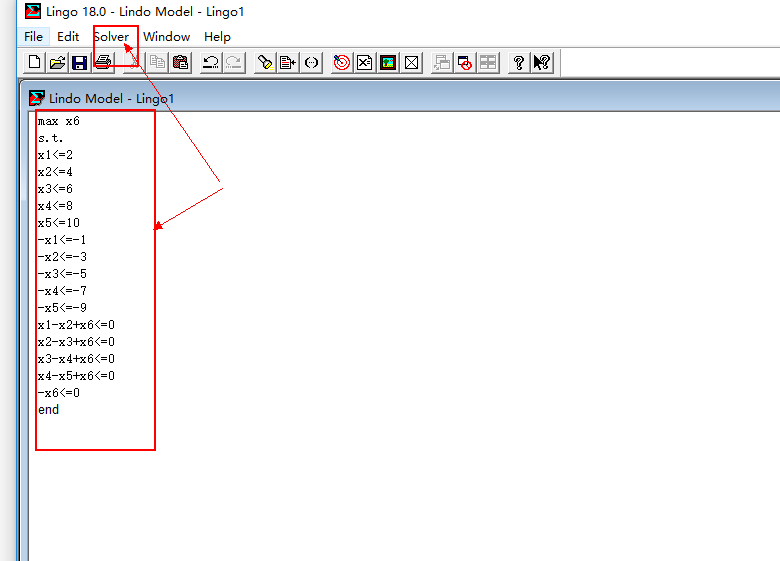在编辑框输入目标函数和约束条件
然后再点击Solver-solve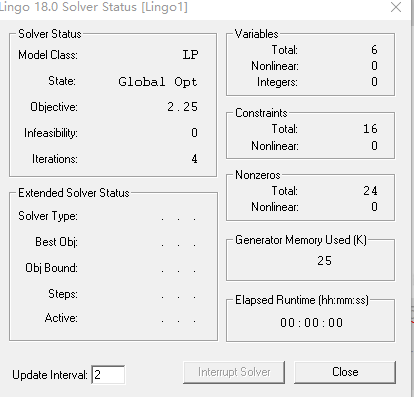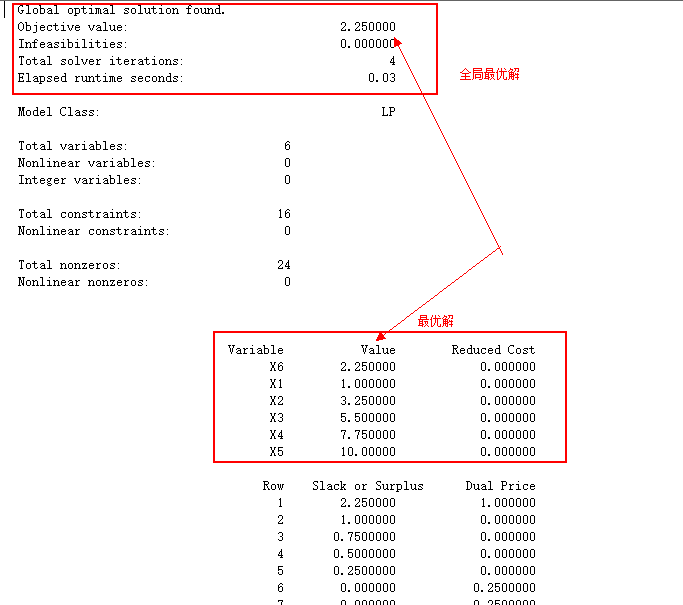Lingo的写法：
1.目标函数 max,min
2.约束：以s.t.或st 或subject to 开头
约束：全部转成标准型！
所有变量都要写上约束,它不会默认说所有变量都是非负，大于等于0也到自己表达出来
所有约束结束 以end 结束
3.整数规划：在end的下一行添加gint 变量名；表示某个变量取值为整数
0/1 规划：int 变量名
特定区间的整数规划：例如[3,8]的整数规划。
约束：
···
x 1 ≤ 8 x_1\leq8
− x 1 ≤ − 3 -x_1\leq-3
e n d end
g i n t   x 1 gint \space x_1

展开全文• 然后, 通过拉格朗日乘子将集合中的复杂约束引入所导出的整数线性规划问题的目标函数中.因为约束系数矩阵是全幺模矩阵, 所以这类整数线性规划问题能用单纯形法容易地求解, 从而可在求解线性规划问题的迭代过程中求出...
• 计算机仿真——线性规划的MATLAB实现 线性规划(Linear Programming Problem:LPP)是优化现实生活中遇到的问题。其数学模型常常由目标函数和约束条件组成。目标函数可以使求最大值，也可以是求最小值，约束条件的不...

## 计算机仿真——线性规划的MATLAB实现

线性规划(Linear Programming Problem:LPP)是优化现实生活中遇到的问题。其数学模型常常由目标函数和约束条件组成。目标函数可以使求最大值，也可以是求最小值，约束条件的不等号可以使小于号也可以是大于号。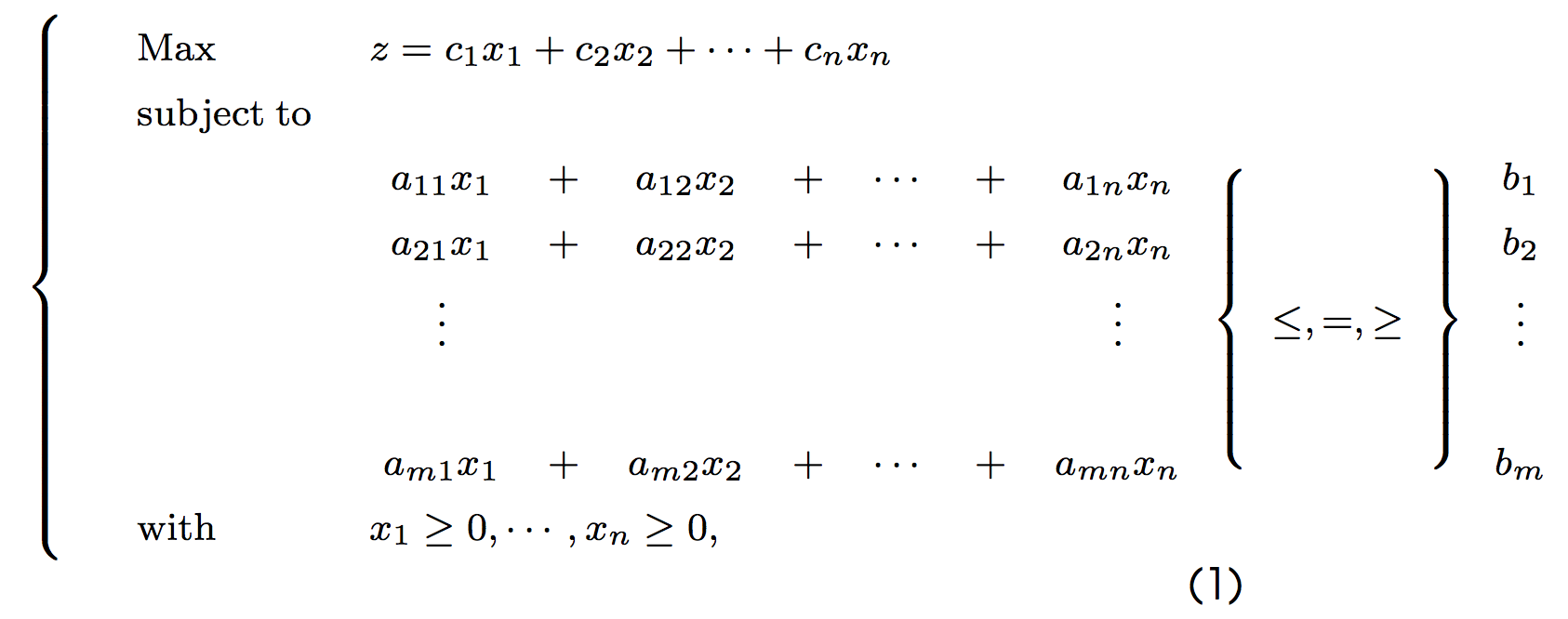为了避免以上形式多样性带来的不便，Matlab中线性规划的标准模为：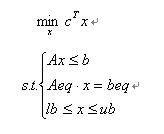基本函数形式为linprog(c,A,b)，它的返回值是向量x的值。还有其它的一些函数调用形式（见附表），如：
[x,fval]=linprog(c,A,b,Aeq,beq,LB,UB,x0,OPTIONS)
其中fval返回目标函数的值，LB和UB为变量x的下界和上界，x0时x的初始值，OPTIONS是控制参数。
【问题提出】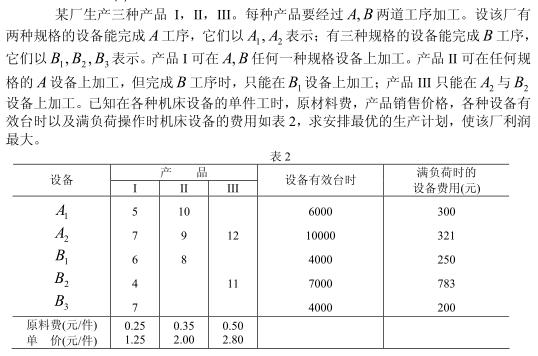【问题分析】
对产品I来说，设A1、A2完成A工序的产品分别为x1,x2件，转入B工序时，以B1、B2、B3完成B工序的产品分别为x3、x4、x5件；对产品II来说，设A1、A2完成A工序的产品为x6，x7件，转入B工序时，以B1完成B工序的产品为x2件；对产品III来说，设以A2完成A工序的产品为x9件，则以B2完成B工序的产品也为x9件。有由上述条件可得数学模型：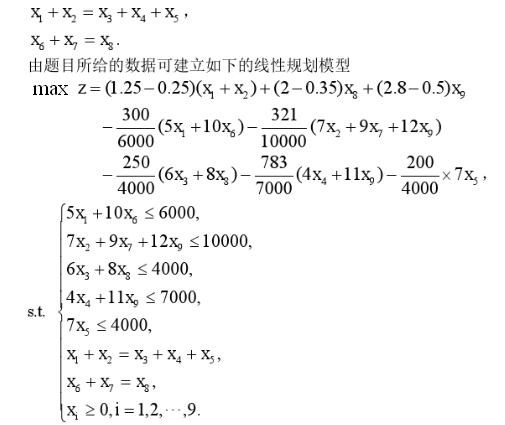【MATLAB程序】
c=zeros(1,9); %目标函数系数c初始化
s=sym(’(1.25-0.25)(x1+x2)+(2-0.35)x8+(2.8-0.5)x9-(5x1+10x6)/20-321(7x2+9x7+12x9)/10000-250(6x3+8x8)/4000-783*(4x4+11x9)/7000-7x5/200’);
s1=simplify(s); %目标函数化简为标准型
s2=coeffs(s1); %获得目标函数的系数c向量
s3=double(s2); %将符号性矩阵转换为数值型矩阵
c(1)=s3(1);c(2)=s3(2);c(8)=s3(3);c(9)=s3(4);c(6)=s3(5);c(7)=s3(6);c(3)=s3(7);c(4)=s3(8);c(5)=s3(9);
A=[5,0,0,0,0,10,0,0,0;0,7,0,0,0,0,9,0,12;0,0,6,0,0,0,0,8,0;0,0,0,4,0,0,0,0,11;0,0,0,0,7,0,0,0,0]; %约束不等式
b=[6000;10000;4000;7000;4000];
Aeq=[1,1,-1,-1,-1,0,0,0,0;0,0,0,0,0,1,1,-1,0]; %约束方程
beq=[0;0];
x=linprog(-c,A,b,Aeq,beq,zeros(9,1)) %线性规划求解算法，返回最优解对应的x值
fprintf(‘The most profit is Value=%.5f\n’,c
x); %求出最优解下的最大利润
【运行结果】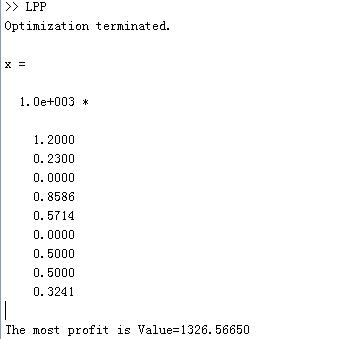附表
LINPROG Linear programming.
X=LINPROG(f,A,b) attempts to solve the linear programming problem:

         min f'*x    subject to:   A*x <= b
x

X=LINPROG(f,A,b,Aeq,beq) solves the problem above while additionally
satisfying the equality constraints Aeq*x = beq.

X=LINPROG(f,A,b,Aeq,beq,LB,UB) defines a set of lower and upper
bounds on the design variables, X, so that the solution is in
the range LB <= X <= UB.  Use empty matrices for LB and UB
if no bounds exist. Set LB(i) = -Inf if X(i) is unbounded below;
set UB(i) = Inf if X(i) is unbounded above.

X=LINPROG(f,A,b,Aeq,beq,LB,UB,X0) sets the starting point to X0.  This
option is only available with the active-set algorithm.  The default
interior point algorithm will ignore any non-empty starting point.

X=LINPROG(f,A,b,Aeq,beq,LB,UB,X0,OPTIONS) minimizes with the default
optimization parameters replaced by values in the structure OPTIONS, an
argument created with the OPTIMSET function.  See OPTIMSET for details.
Options are Display, Diagnostics, TolFun, LargeScale, MaxIter.
Currently, only 'final' and 'off' are valid values for the parameter
Display when LargeScale is 'off' ('iter' is valid when LargeScale is 'on').

[X,FVAL]=LINPROG(f,A,b) returns the value of the objective function at X:
FVAL = f'*X.

[X,FVAL,EXITFLAG] = LINPROG(f,A,b) returns an EXITFLAG that describes the
exit condition of LINPROG. Possible values of EXITFLAG and the corresponding
exit conditions are

1  LINPROG converged to a solution X.
0  Maximum number of iterations reached.
-2  No feasible point found.
-3  Problem is unbounded.
-4  NaN value encountered during execution of algorithm.
-5  Both primal and dual problems are infeasible.
-7  Search direction became too small; no further progress can be made.

[X,FVAL,EXITFLAG,OUTPUT] = LINPROG(f,A,b) returns a structure
OUTPUT with the number of iterations taken in OUTPUT.iterations, the type
of algorithm used in OUTPUT.algorithm, the number of conjugate gradient
iterations (if used) in OUTPUT.cgiterations, and the exit message in
OUTPUT.message.

[X,FVAL,EXITFLAG,OUTPUT,LAMBDA]=LINPROG(f,A,b) returns the set of
Lagrangian multipliers LAMBDA, at the solution: LAMBDA.ineqlin for the
linear inequalities A, LAMBDA.eqlin for the linear equalities Aeq,
LAMBDA.lower for LB, and LAMBDA.upper for UB.

NOTE: the LargeScale (the default) version of LINPROG uses a primal-dual
method. Both the primal problem and the dual problem must be feasible
for convergence. Infeasibility messages of either the primal or dual,
or both, are given as appropriate.  The primal problem in standard
form is
min f'*x such that A*x = b, x >= 0.
The dual problem is
max b'*y such that A'*y + s = f, s >= 0.

展开全文matlab
• ## 线性规划对偶问题

万次阅读 多人点赞 2021-04-28 23:49:31
线性规划对偶问题线性规划及单纯形法〇． 前言一． 对偶问题的提出二． 对称形式下对偶问题的一般形式三． 非对称形式的原-对偶问题关系四． 对偶问题的基本性质五． 对偶问题的单纯形法描述六． 影子价格七． 利用...

# 线性规划及单纯形法

线性规划是最优化问题的一种特殊情形，实质是从多个变量中选取一组合适的变量作为解，使得这组变量满足一组确定的线性式（约束条件），而且使一个线性函数（目标函数）达到最优

## 〇． 前言

不少人对线性规划问题还只有初高中“图解法”的印象。能使用图像直观明了地解题自然是一种好方法，相信很多人都认为一种好方法够解决需要解决的问题不就足矣了吗。其实我也不例外。这也是我开始接触时的疑惑。但学了一阵子后，我思索了，确实，相比较几何和组合推理，代数学确实在非数学专业的学生看来不够讨喜，尽管代数学相当强大、相当普适……单纯形法就是代数的一个小小缩影，它似乎表面上让线性规划问题变得比“图解法”要复杂得多，但是其超强的普适性着实迷人，我们用计算机来解决线性规划问题可能只要一句编程，但其背后的数学原理却如此丰富。我们光线性规划问题就学了9周（还没上完），可见它背后的数学文化还是相当值得深挖研讨的……<其实为了应试【捂嘴】>
因为单纯形法经过本人预习复习后已经有些开窍了，并成功用大M法解决了我的大作业，所以在此不过多赘述，有兴趣可以去了解【我更倾向于你没兴趣，看完你就知道了】不过鉴于这周要测验，而对偶问题还是迷迷糊糊的我决定开始摸鱼做笔记<说白了就是应试>，希望敲完这篇，我可以把线性规划的对偶理论啃下来吧……<说白了还是为了应试>

果然，第一次还是给了数学……哎嘿~~

## 一． 对偶问题的提出

无论从理论或是实践角度，对偶理论是线性规划中的一个最重要和有趣的概念。支持对偶理论的基本思想是，每一个线性规划问题都存在一个与其对偶的问题，在求出一个问题解的时候，也同时给出了另一问题的解。下面通过实际例子看待对偶问题的经济意义。
<书本上的例子实在是过于晦涩难懂，于是在网上摘了一点好理解的“栗子”……>

【例一】
某工厂用甲乙两种资源生产A、B、C、D四种产品，现有资源数、单位产品所需资源数以及单位产品可获得利润如下表所示。问如何组织生产能够使得利润最大？根据题意列出的线性规划不等式是这样的（大家不用去推出这个公式，目的在于和下文的对偶问题公式形成对比）：但是现在如果从另一个角度考虑问题。假设该厂不生产A、B、C、D四种产品，而是将甲、乙两种资源出租给其他单位，其原则是：识别的单位愿意租，又使本单位获利不低于原利润。问如何给甲、乙两种资源定价最合理？

根据题意列出的线性规划不等式是这样的：可以发现，两个问题下的线性规划公式很相似（具体的如何转换会在下文予以说明）。那么两个问题具有什么样的实际意义呢？可以考虑该厂的目的现在是想要出租资源但是要保证价格不低于资源变成产品所带来的收益。也就是说第二个问题所求出来的最小(优)值应该是第一个问题求出的最大(优)值，换句话说我们可以通过原问题的对偶问题的最优值来获得原问题的最优值，但为什么要这样做呢？直接用原问题来求得最优值不可以吗？这就是我们第二个问题所涉及的了。

【例二】
① ：仔细对比上图两种式子可以发现，图一中的变量较多而且约束条件较少，相信大家都做过线性规划的问题，不难发现变量越少，约束条件越多对于我们的求解就越有利。这里也是这个道理，通过将原问题转换成为其对偶问题，可以使得更加有利于我们求解线性规划问题，并且从问题一的解答中我们了解到两种问题“本是同根生”，所以对偶问题其实是有利于我们计算复杂线性规划问题的一种"辅助"方式。但是，对偶问题一定比原问题变量要少吗？并不是这样的，但是我们可以非常容易的判断出该问题的对偶问题会不会更简单，这个方法涉及到对偶问题的转换，我们在第三个问题中进行解答。
② ：其实有时不仅仅是为了减少变量的个数，有的问题甚至必须要通过转换称为对偶问题才能够解决(博主目前的水平下，非数学专业)，比如为了将原式化成标准式时会出现(不)等式右端出现负数的情况，这时如果仅用单纯形法是不能够解决的，因此从这个角度来看，为应对考试对偶问题是必须要学习的。

【例三】
接下来我们将进入实战，直接用实例来讲解原问题的对偶问题是如何化成的。首先我们以下面这个线性规划问题为例：1. 对偶问题的目标函数和原问题是相反的，原问题是min则对偶问题为max。并且变量的个数也会发生改变，系数是原问题不等式右端的b值(仅仅是化为对偶问题是不需要将原问题化作标准式的)。根据以上得出目标函数：2. 接下来是写约束条件，约束条件的书写是最容易出错的地方。我们先写等式的左端，对偶问题等式的左端是根据原问题等式左端竖着来写的；等式的右端就是直接用原问题目标函数中的系数(先不考虑符号),也就是看如下画红框的部分：3. 根据原问题竖着的系数来作为对偶问题每个等式中变量的系数；原问题目标函数的系数，可以得出如下（先仔细看下红框里的数据是如何得到的）：4. 接着是最为重点的约束条件中的符号和变量的范围符号，这两点是根据如下来进行变换：解释： 根据max类型写min类型的变量符号时，要根据max的约束条件符号，并且与之相反；写min的约束条件符号时，要根据max类型的变量符号，并且与之相同。反之亦然。另外无约束对应的是‘ = ’。最终得到：至此，我们已经讲完了对偶问题的转换方法，下面再举一个max类型转换成min类型的例子，大家可以对照练习加深印象。<因为壳子比较菜又比较摸鱼还不会举“栗子”，所以第一部分的“栗子”都是从优秀博主家摘的……后面有走心原创哦~~>

## 二． 对称形式下对偶问题的一般形式

<你们最爱的 纯数学理论来辣~~~>
定义：满足下列条件的线性规划问题称为具有对称形式：其变量均具有非负约束，其约束条件当目标函数求极大时均取“≤”号，当目标函数求极小时均取“≥”号。
对称形式下线性规划原问题的一般形式为：用yi(i=1,…,m)代替第i种资源的估价，则其对偶问题的一般形式为：用矩阵形式表示，对称问题的线性规划问题的原问题为：其对偶问题为：上述对偶问题中令w’=-w，可改写为：将上述对称形式下线性规划的原问题与对偶问题进行比较，可以列出如下表的对应关系：如将其作为原问题，并按上表所列对应关系写出它的对偶问题则有：再令z=-z’，则上式可改写为：可见对偶问题的对偶即原问题。因此将表中右端的线性规划问题作为原问题，写出其左端形式的对偶问题。

## 三． 非对称形式的原-对偶问题关系

因为并非所有线性规划问题具有对称形式，故下面讨论一般情况下线性规划问题如何写出其对偶问题。考虑下面例子：
<随便举个栗子啊>
【例四】 写出下述线性规划问题的对偶问题：解：

为了写出对偶问题，思路是先将其转化成对称形式，再按上表的对应关系来写。因例中目标函数为max，故约束条件应变换为“≤”号，所有的变量均应为≥0.为此：
<emmm…好像忘了给方程标号了…随手就标，养成好习惯><好了，go on…>

（1）约束条件b两端乘上“-1”；
（2）将约束条件c先等价转换为x1+x2+x3≤4和x1+x2+x3≥4，再变换为x1+x2+x3≤4和-x1-x2-x3≤-4；
（3）令x2’=-x2，所以x2’≥0；
（4）令x3=x3’-x3’’，其中x3’≥0，x3’'≥0。

由此【例四】可变换成具有如下对称形式的线性规划问题：令对应上述4个约束条件的对偶变量分别为y_1,y_2’,y_3’,y_3^’’，按表的对应关系写出其对偶问题为：再令y2=y2’,y3=y3’-y3’’,将第三四个约束条件改为一个等式-5y1-6y2’+y3=3，于是有：将上述对偶问题同【一】的原问题对比发现，无论对称或非对称的线性规划问题在写出其对偶向题时，表中前4行的对应关系都适用，区别的只是约束条件的形式与其对应变量的取值。根据本例中约束和变量的对应关系，下面将对称或不对称线性规划原问题同对偶问题的对应关系，统一归纳为下表所示形式：<看到这里是不是感jio要吐了……而这才只到如何写线性规划的对偶问题，你还没解呢……急啥，快到性质了……>

## 四． 对偶问题的基本性质

<这里才是你真正应该感jio到要吐的地方…>
<还是决定手写，这里敲公式多半会哭死……>

1. 弱对偶性·弱对偶性的推论：
（1） 原问题任一可行解的目标函数值时其对偶问题目标函数值的下界；反之对偶问题任一可行解的目标函数值是其原问题目标函数值的上界。<有点儿绕，但不是不好理解>
（2） 如原问题有可行解且目标函数值无界（具有无界解），则其对偶问题无可行解；反之对偶问题有可行解且目标函数值无界，则原问题无可行解（注意：本点性质的逆不成立，当对偶问题无可行解时，其原问题或具有无界解或无可行解，反之亦然）。
（3） 若原问题有可行解而其对偶问题无可行解，则原问题目标函数值无界；反之对偶问题有可行解而其原问题无可行解时，其对偶问题的目标函数值无界。

2. 最优性3. 强对偶性（或称对偶原理）

若原问题及其对偶问题均具有可行解，则两者均具有最优解，且它们最优解的目标函数值相等。

由于两者均有可行解，根据弱对偶性的推论（1），对原问题的目标函数值具有上界，对偶问题的目标函数值具有下界，因此两者均具有最优解。当原问题为最优解时，其对偶问题的解为可行解，且有z=w，由最优性知，这时两者的解均为最优解。

4. 互补松弛性5.基解互补性
原问题及其对偶问题之间存在一对互补的基解，其中原问题的松弛变量对应对偶问题的变量对偶问题的剩余变量对应原问题的变量.这些互相对应的变量如果在一个问题的解中是基变量则在另一个问题的解中是非基变量；将这对互补的集解分别带入原问题和对偶问题的目标函数中有 z = w

## 五． 对偶问题的单纯形法描述

<听嗦考试必考……但其实我并不熟悉，慌~~>
既然是只菜狗，那就参考一下大佬的吧：

参考链接：
对偶问题的单纯形法

## 六． 影子价格

首先，我们先要认识到互补松弛性的作用：
① 简化求对偶问题最优解过程 : 已知一个线性规划问题的最优解 , 可以 简化求另外一个问题最优解的过程 , 避免使用两次单纯形法求解 ;
② 影子价格问题 : 使用互补松弛定理可以进行一些 经济解释 , 如影子价格问题 ;
影子价格 是 对偶问题的 经济解释 ;影子价格是对偶问题的变量值
<懒了，不想举“栗子”了，有关边际价格的“栗子”还挺多的，有兴趣可以在*“参考”*里摘摘>

## 七． 利用Matlab解决线性规划问题

因为毕竟是计算机系的在读小学生，那就提一嘴实战应用咯。因为linprog这个函数比较常用，也是建模赛事中最最最基础的数学模型了，所以对于大家也不陌生。不过你想要用好它可不止单纯会敲一个linprog那么easy，至少你要知道线性规划的标准型，这样才能套对参数，合理求解线性规划问题。
所以简单截个图：<就是懒？不（shi）是（de）>### 八． 参考

链接: https://blog.csdn.net/qq_43539633/article/details/109150749
链接: https://blog.csdn.net/PursueLuo/article/details/112251520
链接: https://blog.csdn.net/weixin_43848054/article/details/105748797
链接: https://blog.csdn.net/shulianghan/article/details/112096559
《运筹学教程》 胡运权 郭耀煌

展开全文• 《运筹学》_习题_线性规划部分练习题及_答案.pdf
• Python之建模规划篇--非线性规划线性规划基本介绍线性规划与非线性规划的区别非线性规划的Matlab解法Python 解决非线性规划1、等式约束下的拉格朗日乘子法2、Python实现对带约束的非线性规划求解Python编程实现...python 算法
• 线性规划与计算复杂性简介PPT学习教案.pptx
• ## matlab线性规划

千次阅读 2022-01-14 22:32:36
一、线性规划 二、问题分类 三、实际分析 四、总结 一、线性规划 1.1简介 在人们的生产实践中，经常会遇到如何利用现有资源来安排生产，以取得最大经济 效益的问题。此类问题构成了运筹学的一个重要分支—...matlab 开发语言 算法
• 针对流域环境系统中存在的不确定性与复杂性问题，运用强化区间线性规划（EILP）方法，建立流域环境系统管理EILP优化模型，并将其应用于四川省邛海流域管理中。EILP作为区间线性规划（ILP）和改进区间线性规划（MILP...
• (同步个人博客http://sxysxy.org/blogs/78 到csdn…可以到 https://github.com/sxysxy/math/tree/master/simplex 下载本文的markdown文件和代码等相关资料线性规划简介:线性规划(Linear Programming, LP)，是运筹学...数学 算法
• 粒子群优化算法(PSO)与其他演化算法相似,也是基于群体的。...对非线性规划例子的实例计算表明,该算法稳定性好,简单容易实现而又功能强大,易于掌握,对于多维非线性、复杂问题的求解具有普遍适用性。
• 最优化设计：第7章-约束问题的非线性规划方法.ppt设计
• - 矩阵对策的概念, 提出一种求解支付为梯形模糊数的矩阵对策线性规划方法. 该方法中局中人双方的对策值始终相同, 且此对策值的任意??- 截集的上下界和局中人的最优策略容易通过求解导出的4 个线性规划问题获得, 特别...
• 线性规划说明 什么是线性规划？ 想象一下，您有一个线性方程组和不等式系统。这样的系统通常有许多可能的解决方案。线性规划是一组数学和计算工具，可让您找到该系统的特定解，该解对应于某些其他线性函数的最大值...python 算法 数据挖掘 django
• 思路是将复杂约束引入到目标函数作为罚项, 得到一个松弛整数线性规划问题. 因为约束系数矩阵是全幺模矩阵, 松弛问题可以通过线性规划很快地求解. 拉格朗日乘子的调整用罚函数的方法很容易计算. 数值实验表明提出的...
• 本文的内容介绍了当今制造业公司普遍采用的定量技术，即线性规划及其对生产计划决策的后续影响。 基于一致意见的结果表明，定量分析模型和工具的“最适合”应用程序可以消除生产和计划决策过程的复杂性，从而实现...
• 线性整数规划的遗传算法 Matlab 程序附图 通常非线性整数规划是一个具有指数复杂度的 NP 问题如果约束较为复杂 Matlab 优化工具箱和一些优化软件比如 lingo 等常常无法应用即使能应用也不能给出一 个较为令人满意...
• 针对问题一：对偶问题在生活中非常常见，可以肯定地说，每一个线性规划问题都存在一个与之对应的对偶问题。其实对偶问题最后的求解虽然和原问题在含义上是不相同的，但是却有某种联系可以将他们连接起来。列举一个书...算法
• ## 线性规划算法详解

万次阅读 多人点赞 2018-07-29 15:41:05
线性规划 首先什么是线性规划，大致的定义我总结为在线性的目标和约束中，找出一个最优解。 举个例子：  M1和M2两种原料用于生产内外墙涂料，M1日最大可用量24吨，M2日最大可用量为6吨，外墙涂料每吨需要6吨M1，...算法
• 针对具有不同维数非线性节点的非线性耦合复杂动态网络, 首先给出了它的模型和实现同步的假设; 然后基于不变流形给出了该类复杂网络同步的定义, 并设计了分散动态补偿控制器, 提出了同步方案; 最后运用Lyapunov 稳定...
• 由于传统模型无法准确描述其地质状况的复杂性,所以在建立属性模型时,采用改进后的加权线性规划法对变差函数进行拟合,随后对地质储量进行复算。研究表明,改进后的加权线性规划法能够显著提高变差函数拟合精度,建立的...
• ## matlab中的线性规划

万次阅读 多人点赞 2019-06-26 13:20:49
matlab中的线性规划 版权声明：该篇由特慢的一七原创，转载请联系创作者。 目前本人基于matlab做了一些建模入门的基础教程，可以在这里分享给大家， 昨日推送：matlab中的线性规划 近期来，好多童鞋面临着数学建模这...matlab linprog
• 这是线性规划的标准型： 这么看未免有些抽象，我们将在代码中逐步了解 1.我们先来看一个题目： 先导入库函数： fromscipyimportoptimize importnumpyasnp 之后，范围限定，注意，系统默认是小于等于且求...python
• matlab的toolbox中没有提供0-1整数非线性规划的求解工具，《高等应用数学问题的matlab求解》中介绍了荷兰Groningen大学的bnb20工具可以进行大部分整数、非整数、混合类的线性、非线性优化问题的求解。文件:bnb20_for...
• 文章目录第一章 线性规划§1 线性规划1.1 线性规划的实例与定义1.2 线性规划的 Matlab 标准形式1.3 线性规划问题的解的概念1.4 线性规划的图解法1.5 求解线性规划的 Matlab 解法 （练习）1.6 可以转化为线性规划的...
• MATLAB教学视频，数学建模与数值计算类：本期视频时长约65分钟，首先通过简单的零食购买问题，引导出线性规划问题的...然后深入讲解复杂的生产分配问题，建立线性规划数学模型并详细讲解linprog函数的高级调用格式。matlab...